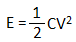Hey, in this article, we are going to know when the capacitor will store more energy series or parallel connection. This article will increase your knowledge about the basics of capacitors. If you want to be a genius then first clear your basic knowledge in electrical engineering. However, let's go to know.

The capacitor is a passive device that can store electrical energy in the form of charge. The energy storing formula of a capacitor is,here the C = Capacitance
V = Applied Voltage across the capacitor

So the storing of electrical energy depends upon the capacitance value of the capacitor and the square of the applied voltage.

In series connection of 4 nos capacitors, the total capacitance value will be,In parallel connection of 4 nos capacitors, the total capacitance value will be,So it is cleared that in parallel connection capacitance value will be more.

As the energy-storing depends upon the capacitance value and voltage, the capacitor will store much more energy in parallel connection than the series connection for the same applied voltage level because in parallel connection capacitance value is more.

Let's clarify this with an example,

Suppose we take 4 nos of identical capacitors which are rated 4 farads, 4 volts.

In series connection, the energy store,In parallel connection, the energy store,So, now you may understand, in parallel connection, the capacitor can store how much energy than the series connection.

Now, if we think practically when a number of capacitors are connected in parallel, all of them will get the same voltage so each one will store energy as per their capacitance value and the net energy storage will be the sum of all the individual energy storages.

When a number of capacitors are connected in series, all of them will not be got the same voltage because the voltage will be drop depending upon the capacitor value distribution. So they not able to charge fully or cannot store maximum energy.

So, the conclusion is Capacitor will store more energy in parallel connection than the series connection.

Thank you for visiting the website. keep visiting for more updates.

When Capacitor store more Energy Series or Parallel connection?Reviewed by Author on March 12, 2020 Rating: 5